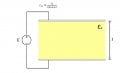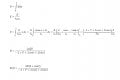# Capacitor with nonconstant dielectric

#### MB4646

Joined Feb 9, 2018
12
I am a student of electrical engineering and a couple of weeks ago, I had got the following homework to do:
I have a capacitor with dielectric material in it. But dielectricity of this material is not constant, it is chanaging with function =0/(cos+1).
They are asking me, to get the formula for electrical field which is chanaging with the distance from one side of capacitor.
I have already tried to solve this, but my professor said it is wrong and he would not tell me why. So I am asking you for help.
I am also attaching the scketch of the problem and my attempt solving it..

#### Bordodynov

Joined May 20, 2015
2,916
V=const !
E=V/L/epsilon
En=V/l/epsilon_n

#### WBahn

Joined Mar 31, 2012
26,398
It would help if you gave some indication of the coordinate system (which direction is 'x' and where is x=0) and of the scale dimension for l compared to the wavelength of the dielectric variation.

#### MB4646

Joined Feb 9, 2018
12
V=const !
E=V/L/epsilon
En=V/l/epsilon_n
I would appreciate if you could explain what you have written.

#### MB4646

Joined Feb 9, 2018
12
It would help if you gave some indication of the coordinate system (which direction is 'x' and where is x=0) and of the scale dimension for l compared to the wavelength of the dielectric variation.
x=0 at upper plate of capacitor, and goes perpendicular on plate to the lower plate. The distance between plates (l) is 5mm. Isn't dielectric variation only in alternating electric field?

#### WBahn

Joined Mar 31, 2012
26,398
x=0 at upper plate of capacitor, and goes perpendicular on plate to the lower plate. The distance between plates (l) is 5mm. Isn't dielectric variation only in alternating electric field?
The dielectric is a physical medium. Imagine taking a bunch of thin sheets of different materials, each having a slightly different dielectric constant, and then stacking them up so that the dielectric constant changed as you went up the stack. You could create any profile you wanted (within reason).

#### MB4646

Joined Feb 9, 2018
12
The dielectric is a physical medium. Imagine taking a bunch of thin sheets of different materials, each having a slightly different dielectric constant, and then stacking them up so that the dielectric constant changed as you went up the stack. You could create any profile you wanted (within reason).
But if I write the sum of all fields in those sheets (so I get voltage) and than I write it as integral, I get the same one as I had in the first place.

#### Bordodynov

Joined May 20, 2015
2,916
I would appreciate if you could explain what you have written.
If you substitute the value of Epsilon in my last formula, you will get an answer. And no integrals are needed.
I would do it for you, but the rules of the forum do not allow. The learning process is important.

#### Bordodynov

Joined May 20, 2015
2,916
Yes.
V is the voltage applied to the plates.

#### MB4646

Joined Feb 9, 2018
12
Yes.
V is the voltage applied to the plates.
What about "l", is it constant (5mm) or is it chanaging (so it is the same like x in cos function)?

#### Bordodynov

Joined May 20, 2015
2,916

#### MB4646

Joined Feb 9, 2018
12
l=L=const
Thank you very much. One more question: How did you get this equation? Is it standard for this kind of capacitor? In which cases can we use it?

#### Bordodynov

Joined May 20, 2015
2,916
MB4646, I am sorry.
E=Q/(epsilon0*epsilon*S), the fundamental formula 1
(V=Q/C, C=(epsilon0*epsilon*S)/d (d=L) --> E=V/d)
Let's select a small section with the number "i". Its length is dX and the width of W
Ei=Qi/(epsilon0*epsilon*S)=Qi/(epsilon0*epsiloni*W*dX)
Ci=(epsilon0*epsiloni*W*dX)/d, the fundamental formula 2
Qi=V*Ci, the fundamental formula 3
Qi=V*(epsilon0*epsiloni*W*dX)/d ==>
Ei=(V*(epsilon0*epsiloni*W*dX)/d) /(epsilon0*epsiloni*W*dX)=V/d=V/L=const

Once again I apologize for the misleading!

Last edited:

#### MB4646

Joined Feb 9, 2018
12
MB4646, I am sorry.
E=Q/(epsilon0*epsilon*S), the fundamental formula 1
(V=Q/C, C=(epsilon0*epsilon*S)/d (d=L) --> E=V/d)
Let's select a small section with the number "i". Its length is dX and the width of W
Ei=Qi/(epsilon0*epsilon*S)=Qi/(epsilon0*epsiloni*W*dX)
Ci=(epsilon0*epsiloni*W*dX)/d, the fundamental formula 2
Qi=V*Ci, the fundamental formula 3
Qi=V*(epsilon0*epsiloni*W*dX)/d ==>
Ei=(V*(epsilon0*epsiloni*W*dX)/d) /(epsilon0*epsiloni*W*dX)=V/d=V/L=const

Once again I apologize for the misleading!
So the field is constant?

#### Bordodynov

Joined May 20, 2015
2,916
For greater rigor of my calculations it is necessary to add: We divide the capacitor into a set of parts dX, where dX -> 0. On this part, the dielectric constant can be considered. The area of the plates of a small capacitor is Si = dX * W, W is the width of the capacitor. It is easy to calculate the capacitance of this flat capacitor. If you understand my calculations, you will not ask any more questions.

#### MB4646

Joined Feb 9, 2018
12
For greater rigor of my calculations it is necessary to add: We divide the capacitor into a set of parts dX, where dX -> 0. On this part, the dielectric constant can be considered. The area of the plates of a small capacitor is Si = dX * W, W is the width of the capacitor. It is easy to calculate the capacitance of this flat capacitor. If you understand my calculations, you will not ask any more questions.
Ok I understand that we divided the capacitor in a lot of little sheets. But I do not understand how do I get equation for electrical field (I have to draw graph). Is the formula, you have written before, wrong?

#### Bordodynov

Joined May 20, 2015
2,916
Yes, everything I wrote at the very beginning is a lie. I studied at the university 40 years ago (and even more so in school) and much has forgotten. I rummaged a bit on the Internet and found the formula: E=Q/(epsilon0*epsilon*S).
This is the formula for the strength of a flat capacitor. It includes the charge of the capacitor and the area of the capacitor. The capacitor charge is determined by the voltage on the capacitor and its capacitance. The capacitance of the capacitor is determined by the area of the electrode and the distance between the plates. Using these three relationships, I received that the strength of the field does not depend on the functional dependence of the dielectric permeability on distance.

#### MB4646

Joined Feb 9, 2018
12
Yes, everything I wrote at the very beginning is a lie. I studied at the university 40 years ago (and even more so in school) and much has forgotten. I rummaged a bit on the Internet and found the formula: E=Q/(epsilon0*epsilon*S).
This is the formula for the strength of a flat capacitor. It includes the charge of the capacitor and the area of the capacitor. The capacitor charge is determined by the voltage on the capacitor and its capacitance. The capacitance of the capacitor is determined by the area of the electrode and the distance between the plates. Using these three relationships, I received that the strength of the field does not depend on the functional dependence of the dielectric permeability on distance.
Maybe that's the catch: so graph should be straight horizontal line. But which equation should I use for calculating this field? Would be good enough if I calculate the average value of function for epsilonN on interval [0,5] and use it as it is the same dielectricity in whole volume? Or is there another equation that would let me calculate the field without epsilonN?

#### Bordodynov

Joined May 20, 2015
2,916
I do not understand what you want. I already did everything. I got that on any very small gap (dX-->0) Field constantly. And he wrote what is equal. The intensity of the field was equal to the voltage on the plates, divided by the distance between the plates. Your task is solved!